# Arctangent function

Arctan(x), tan-1(x), inverse tangent function.

### Arctan definition

The arctangent of x is defined as the inverse tangent function of x when x is real (x∈ℝ).

When the tangent of y is equal to x:

tan y = x

Then the arctangent of x is equal to the inverse tangent function of x, which is equal to y:

arctan x= tan-1 x = y

#### Example

arctan 1 = tan-1 1 = π/4 rad = 45°

### Graph of arctan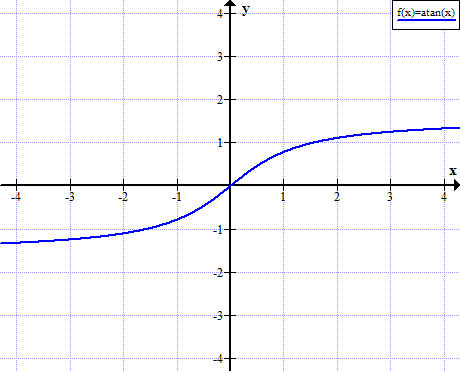### Arctan rules

Rule name Rule
Tangent of arctangent

tan( arctan x ) = x

Arctan of negative argument

arctan(-x) = - arctan x

Arctan sum

arctan α + arctan β = arctan [(α+β) / (1-αβ)]

Arctan difference

arctan α - arctan β = arctan [(α-β) / (1+αβ)]

Sine of arctangent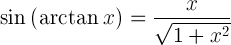Cosine of arctangent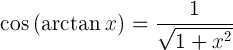Reciprocal argument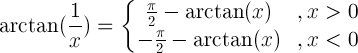Arctan from arcsin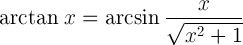Derivative of arctan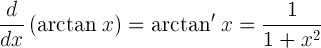Indefinite integral of arctan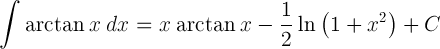### Arctan table

x arctan(x)

arctan(x)

(°)

-∞ -π/2 -90°
-3 -1.2490 -71.565°
-2 -1.1071 -63.435°
-√3 -π/3 -60°
-1 -π/4 -45°
-1/√3 -π/6 -30°
-0.5 -0.4636 -26.565°
0 0
0.5 0.4636 26.565°
1/√3 π/6 30°
1 π/4 45°
3 π/3 60°
2 1.1071 63.435°
3 1.2490 71.565°
π/2 90°